# 12. Overview of Matplotlib

## Introduction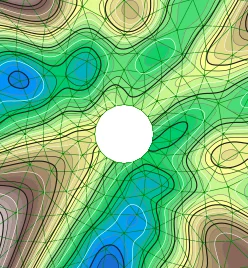Matplotlib is a plotting library like GNUplot. The main advantage towards GNUplot is the fact that Matplotlib is a Python module. Due to the growing interest in python the popularity of matplotlib is continually rising as well.

Another reason for the attractiveness of Matplotlib lies in the fact that it is widely considered to be a perfect alternative to MATLAB, if it is used in combination with Numpy and Scipy. Whereas MATLAB is expensive and closed source, Matplotlib is free and open source code. It is also object-oriented and can be used in an object oriented way. Furthermore it can be used with general-purpose GUI toolkits like wxPython, Qt, and GTK+. There is also a procedural "pylab", which designed to closely resemble that of MATLAB. This can make it extremely easy for MATLAB users to migrate to matplotlib.

Matplotlib can be used to create publication quality figures in a variety of hardcopy formats and interactive environments across platforms.

Another characteristic of matplotlib is its steep learning curve, which means that users usually make rapid progress after having started. The officicial website has to say the following about this: "matplotlib tries to make easy things easy and hard things possible. You can generate plots, histograms, power spectra, bar charts, errorcharts, scatterplots, etc, with just a few lines of code."

Live Python training

Enjoying this page? We offer live Python training courses covering the content of this site.

Enrol here

## A First Example: Line Plot

We will start with a simple graph , which is as simple as simple can be. A graph in matplotlib is a two- or three-dimensional drawing showing a relationship by means of points, a curve, or amongst others a series of bars. We have two axis: The horizontal X-axis is representing the independent values and the vertical Y-axis corresponds to the depended values.

We will use the pyplot submodule of matplotlib. pyplot provides a procedural interface to the object-oriented plotting library of matplotlib.

Its plotting commands are chosen in a way that they are similar to Matlab both in naming and with the arguments.

Is is common practice to rename matplotlib.pyplot to plt. We will use the plot function of pyplot in our first example. We will pass a list of values to the plot function. Plot takes these as Y values. The indices of the list are automatically taken as the X values. The command %matplotlib inline makes only sense, if you work with Ipython Notebook. It makes sure, that the graphs will be depicted inside of the document and not as independent windows:

import matplotlib.pyplot as plt

plt.plot([-1, -4.5, 16, 23, 78, 22, 3])
plt.show()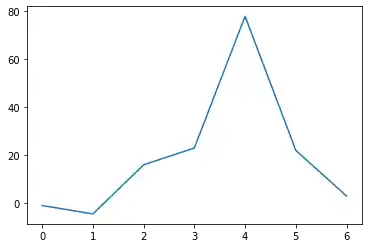What we see is a continuous graph, even though we provided discrete data for the Y values. By adding a format string to the function call of plot, we can create a graph with discrete values, in our case blue circle markers. The format string defines the way how the discrete points have to be rendered.

import matplotlib.pyplot as plt

plt.plot([-1, -4.5, 16, 23, 78, 22, 3], "ob")
plt.show()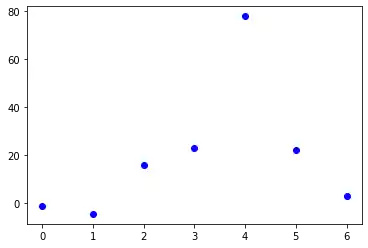With only a little bit of input we were capable in the previous examples to create plots. Yet, the plots missed some information. Usually, we would like to give a name to the 'x' and 'y' values. Furthermore, the whole graph should have a title. We demonstate this in the following example:

import matplotlib.pyplot as plt

days = range(1, 9)
celsius_values = [25.6, 24.1, 26.7, 28.3, 27.5, 30.5, 32.8, 33.1]

fig, ax = plt.subplots()
ax.plot(days, celsius_values)
ax.set(xlabel='Day',
ylabel='Temperature in Celsius',
title='Temperature Graph')

plt.show()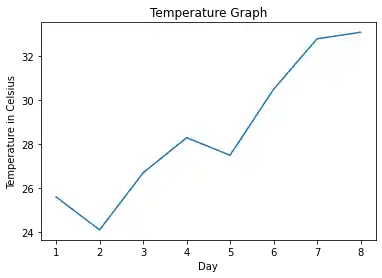## Multiple Plots in one Graph

We saw that the plot function is needed to plot a figure or better the figures, because there may be more than one.

We can specify an arbitrary number of x, y, fmt groups in a one plot function. We will extend our previous temperature example to demonstrate this. We provide two lists with temperature values, one for the minimum and one for the maximum values:

import matplotlib.pyplot as plt

days = list(range(1,9))
celsius_min = [19.6, 24.1, 26.7, 28.3, 27.5, 30.5, 32.8, 33.1]
celsius_max = [24.8, 28.9, 31.3, 33.0, 34.9, 35.6, 38.4, 39.2]

fig, ax = plt.subplots()

ax.set(xlabel='Day',
ylabel='Temperature in Celsius',
title='Temperature Graph')

ax.plot(days, celsius_min,
days, celsius_min, "oy",
days, celsius_max,
days, celsius_max, "or")

plt.show()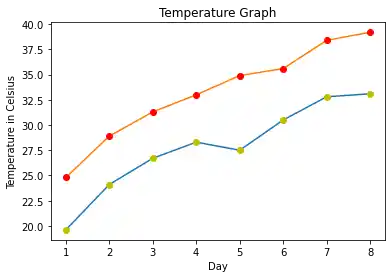We could have used four plot calls in the previous code instead of one, even though this is not very attractive:

import matplotlib.pyplot as plt

days = list(range(1, 9))
celsius_min = [19.6, 24.1, 26.7, 28.3, 27.5, 30.5, 32.8, 33.1]
celsius_max = [24.8, 28.9, 31.3, 33.0, 34.9, 35.6, 38.4, 39.2]

fig, ax = plt.subplots()

ax.set(xlabel='Day',
ylabel='Temperature in Celsius',
title='Temperature Graph')

ax.plot(days, celsius_min)
ax.plot(days, celsius_min, "oy")
ax.plot(days, celsius_max)
ax.plot(days, celsius_max, "or")

plt.show()Live Python training

Enjoying this page? We offer live Python training courses covering the content of this site.

Upcoming online Courses

Enrol here

## Example: Bar Plots

A more formal introduction will follow later in our tutorial on Matplotlib.

import matplotlib.pyplot as plt
import numpy as np
years = [str(year) for year in range(2010, 2021)]
visitors = (1241, 50927, 162242, 222093,
665004, 2071987, 2460407, 3799215,
5399000, 5474016, 6003672)

plt.bar(years, visitors, color="green")

plt.xlabel("Years")
plt.ylabel("Values")
plt.title("Bar Chart Example")

plt.plot()
plt.show()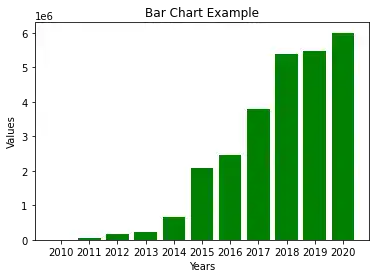## Histograms

import matplotlib.pyplot as plt
import numpy as np

gaussian_numbers = np.random.normal(size=10000)
gaussian_numbers

plt.hist(gaussian_numbers, bins=20)
plt.title("Gaussian Histogram")
plt.xlabel("Value")
plt.ylabel("Frequency")
plt.show()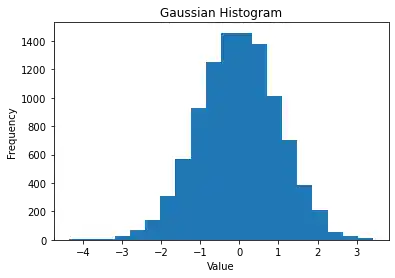Live Python training

Enjoying this page? We offer live Python training courses covering the content of this site.

Enrol here

## Scatter Plots

import matplotlib.pyplot as plt
import numpy as np

x = np.arange(0, 11)

y1 = np.random.randint(2, 7, (11,))
y2 = np.random.randint(9, 14, (11,))
y3 = np.random.randint(15, 25, (11,))

# Markers: https://matplotlib.org/api/markers_api.html

plt.scatter(x, y1)
plt.scatter(x, y2, marker='v', color='r')
plt.scatter(x, y3, marker='^', color='m')
plt.title('Scatter Plot Example')
plt.show()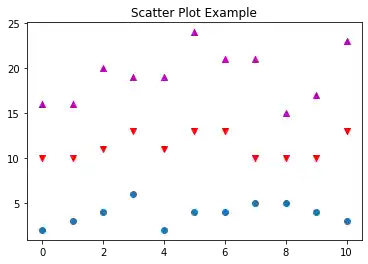## Stack Plots

import matplotlib.pyplot as plt

idxes = [ 1,  2,  3,  4,  5,  6,  7,  8,  9]
y1  = [23, 42, 33, 43,  8, 44, 43, 18, 21]
y2  = [9, 31, 25, 14, 17, 17, 42, 22, 28]
y3  = [18, 29, 19, 22, 18, 16, 13, 32, 21]

plt.stackplot(idxes,
y1, y2, y3)
plt.title('Stack Plot Example')

plt.show()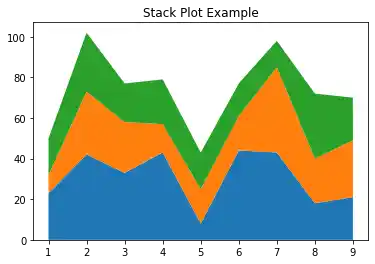Live Python training

Enjoying this page? We offer live Python training courses covering the content of this site.

Enrol here

## Pie Charts

import matplotlib.pyplot as plt

# Pie chart, where the slices will be ordered and plotted counter-clockwise:
labels = 'C', 'Python', 'Java', 'C++', 'C#'
sizes = [13.38, 11.87, 11.74, 7.81, 4.41]
explode = (0, 0.1, 0, 0, 0)  # only "explode" the 2nd slice (i.e. 'Hogs')

fig1, ax1 = plt.subplots()
ax1.pie(sizes, explode=explode, labels=labels, autopct='%1.1f%%',
ax1.axis('equal')  # Equal aspect ratio ensures that pie is drawn as a circle.

plt.title('TIOBE Index for May 2021')
plt.show()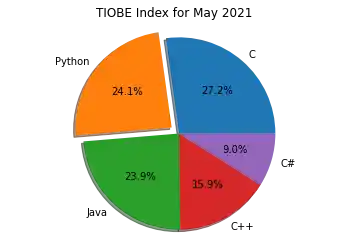import matplotlib.pyplot as plt

# Pie chart, where the slices will be ordered and plotted counter-clockwise:
labels = 'Python', 'C', 'Java', 'C++', 'C#'
sizes = [14.26, 13.06, 11.19, 8.66, 5.92]
explode = (0.1, 0, 0, 0, 0)  # only "explode" the 2nd slice (i.e. 'Hogs')

fig1, ax1 = plt.subplots()
ax1.pie(sizes, explode=explode, labels=labels, autopct='%1.1f%%',
ax1.axis('equal')  # Equal aspect ratio ensures that pie is drawn as a circle.

plt.title('TIOBE Index for March 2022')
plt.show()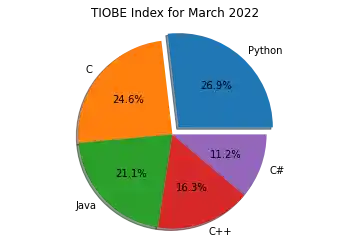# Pie chart, where the slices will be ordered and plotted counter-clockwise:
labels = 'Python', 'C', 'Java', 'C++', 'C#', 'others'
sizes = [14.26, 13.06, 11.19, 8.66, 5.92]
sizes.append(100 - sum(sizes))
explode = (0, 0.1, 0, 0, 0, 0)  # only "explode" the 2nd slice (i.e. 'Hogs')

fig1, ax1 = plt.subplots()
ax1.pie(sizes, explode=explode, labels=labels, autopct='%1.1f%%',
ax1.axis('equal')  # Equal aspect ratio ensures that pie is drawn as a circle.

plt.title('TIOBE Index for March 2022')
plt.show()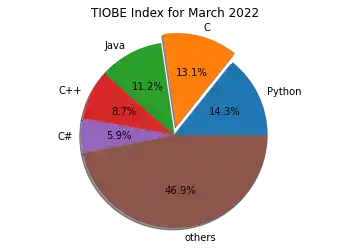Live Python training

Enjoying this page? We offer live Python training courses covering the content of this site.

Upcoming online Courses

Enrol here